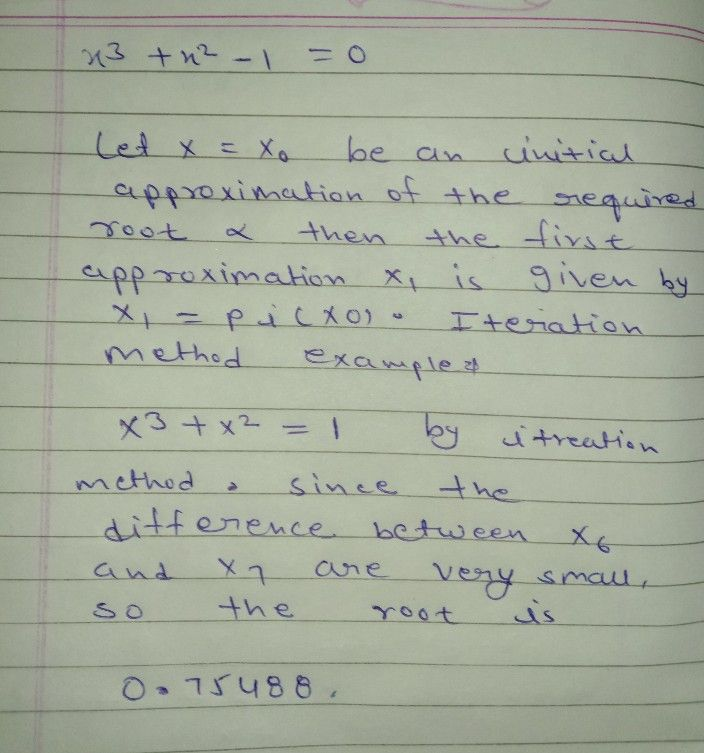Symbol
Problem$10$ the error in the previous stage. we $5ay$ $+==$ linear. i I Isvoint orli Tovo (10 to oulev mumiXBUT $O$ $\square$ M JOI Example $1$ Find a real root of the equation $x^{3}+x^{2}-1=0$ by iteration method. $3.-2-1$ $a\right)$ $1-11$
Other
Search count: 105
SolutionQanda teacher - Anushka